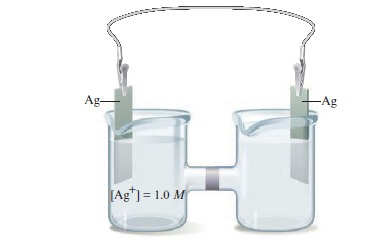# Problem: Consider the concentration cell shown below. Calculate the cell potential at 25°C when the concentration of Ag+ in the compartment on the right is the following.c. 0.10 MFor each case, also identify the cathode, the anode, and the direction in which electrons flow.

###### FREE Expert Solution

Concentration cell → anode and cathode are the same → cell = 0

Modified Nernst equation:

$\overline{){{\mathbf{E}}}_{{\mathbf{cell}}}{\mathbf{=}}{\mathbf{E}}{{\mathbf{°}}}_{{\mathbf{cell}}}{\mathbf{-}}\mathbf{\left(}\frac{\mathbf{0}\mathbf{.}\mathbf{0592}}{\mathbf{n}}\mathbf{\right)}{\mathbf{log}}\frac{\left[\mathrm{anode}\right]}{\left[\mathrm{cathode}\right]}}$

• anodelower concentration
• cathode higher concentration
79% (139 ratings)###### Problem Details

Consider the concentration cell shown below. Calculate the cell potential at 25°C when the concentration of Ag+ in the compartment on the right is the following.

c. 0.10 M

For each case, also identify the cathode, the anode, and the direction in which electrons flow.